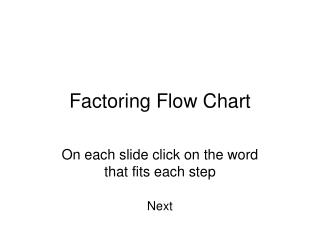DownloadDownload PresentationFactoring Flow Chart

# Factoring Flow Chart

Télécharger la présentation## Factoring Flow Chart

- - - - - - - - - - - - - - - - - - - - - - - - - - - E N D - - - - - - - - - - - - - - - - - - - - - - - - - - -
##### Presentation Transcript

1. Factoring Flow Chart On each slide click on the word that fits each step Next

2. Look for greatest common monomial factorsNext

3. What does your problem look like • Is it a binomial? • Is it a trinomial? • Is it a polynomial with 4 or more terms?

4. Binomial • Is it the difference between two perfect squares? • Yes • No

5. Factor to form(x + c)(x – c)Next

6. Trinomials • Does your trinomial have a leading coefficient of : • One • A number other than one

7. Trinomial with leading coefficient other than one • Is your last sign: • Positive • Negative

8. Last sign positive • Is your middle sign: • Positive • Negative

9. Last sign positive and middle sign positiveUse (ax + c)(bx + d) and check for your middle term • Are one of your factors difference between two perfect squares • Yes • No

10. Last sign positive and middle sign negativeUse (ax - c)(bx - d) and check for your middle term • Are one of your factors difference between two perfect squares • Yes • No

11. Last sign negativeUse (ax + c)(bx - d) and check for your middle term • Are one of your factors difference between two perfect squares • Yes • No

12. Write as a product of two binomials • Is your last sign: • Positive • Negative

13. Last sign positive • Is your middle sign: • Positive • Negative

14. Last sign positive and middle sign positiveUse (x + c)(x + d) and check for your middle term • Are one of your factors difference between two perfect squares • Yes • No

15. Last sign positive and middle sign negativeUse (x - c)(x - d) and check for your middle term • Are one of your factors difference between two perfect squares • Yes • No

16. Last sign negativeUse (x + c)(x - d) and check for your middle term • Are one of your factors difference between two perfect squares • Yes • No

17. Polynomial with 4 or more terms • Group two terms that have a common monomial factor • Factor out a common binomial factor • Ex. Ax + Ay + 3x + 3y • A(x + y) + 3(x + y) • (x + y)(A + 3) • Hint look for oppostites

18. Congratulations you have successfully completed the problem.Would you like to do another one? • Yes • No